You are currently offline. Some features of the site may not work correctly.

# Five color theorem

Known as: Five colour theorem, Five-color map theorem
The five color theorem is a result from graph theory that given a plane separated into regions, such as a political map of the counties of a state… Expand
Wikipedia

## Papers overview

Semantic Scholar uses AI to extract papers important to this topic.
2015
2015
With the help of Neutrosophy and Quad-stage Method, the proof for negation of “the four color theo-rem” is given. In which the… Expand
•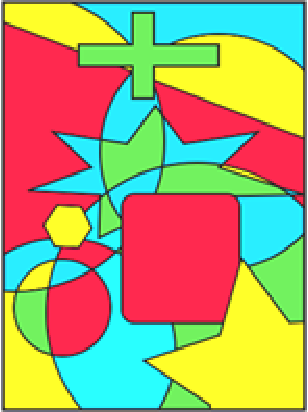•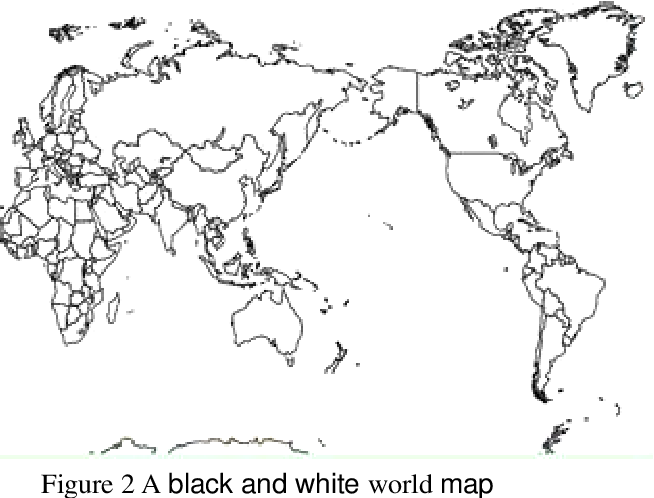2012
2012
In this paper, we will define the necessary concepts to formulate map coloring problems. Then, we will prove Euler’s formula and… Expand
2009
2009
A formal proof has not been found for the four color theorem since 1852 when Francis Guthrie first conjectured the four color… Expand
•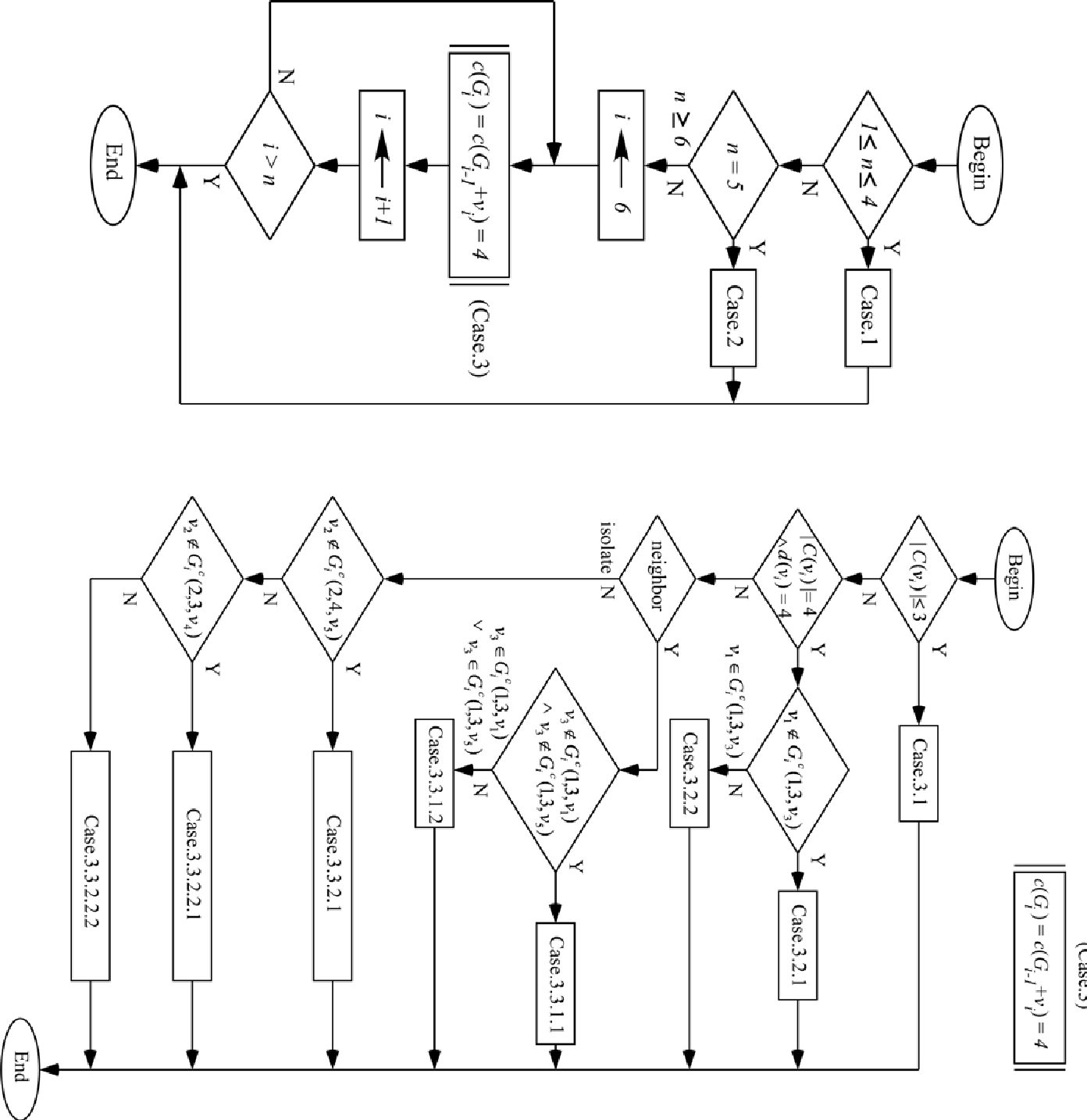2004
2004
• J. Comb. Theory, Ser. B
• 2004
• Corpus ID: 21542117
We prove τodd(G) ≤ 2Vodd(G) for each planar graph G where vodd(G) is the maximum number of edge-disjoint odd cycles and τodd(G… Expand
•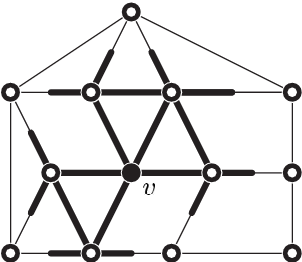•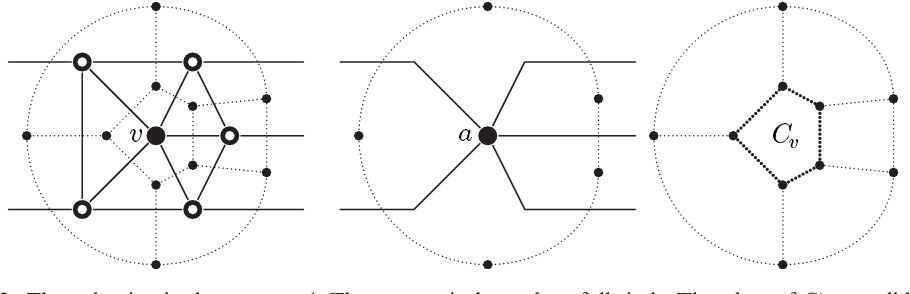•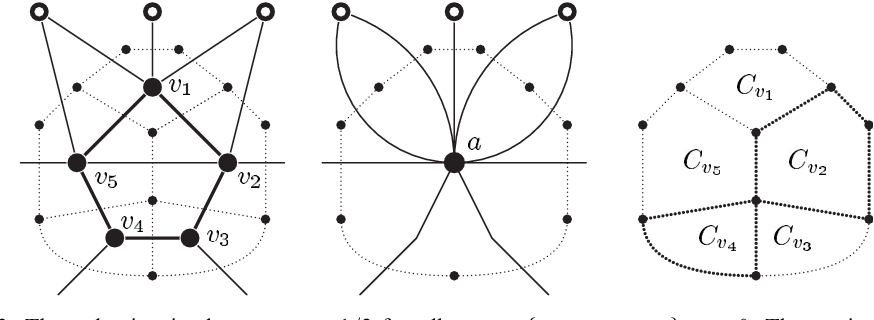•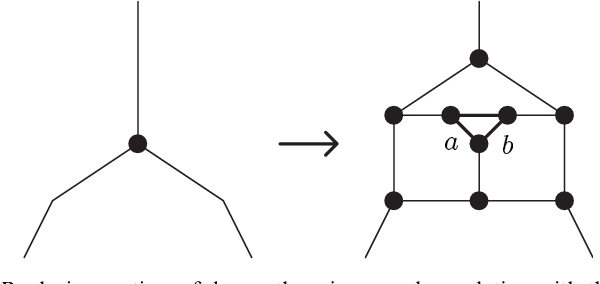2002
2002
Based on a representation of primitive proof objects as λ-terms, which has been built into the theorem prover Isabelle recently… Expand
•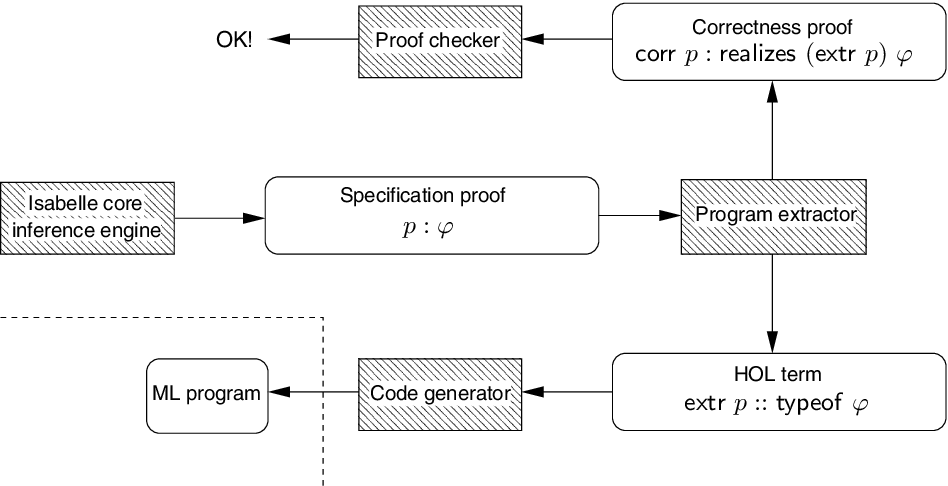Highly Cited
2002
Highly Cited
2002
In this paper, we introduce graph theory, and discuss the Four Color Theorem. Then we prove several theorems, including Euler’s… Expand
2000
2000
• Discret. Math.
• 2000
• Corpus ID: 205874569
Abstract In this note, we show that the edges and faces of any plane graph with maximum degree three can be simultaneously… Expand
•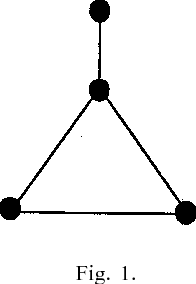1981
1981
• 1981
• Corpus ID: 118009603
1976
1976
1975
1975
Let f be a face of a plane graph G. The Three and Five Color Theorem proved here states that the vertices of G can be colored… Expand